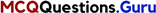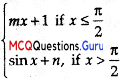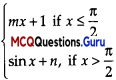# HSSlive: Plus One & Plus Two Notes & Solutions for Kerala State Board

## MCQ Questions for Class 12 Maths Chapter 5 Continuity and Differentiability With Answers PDFMCQ Questions for Class 12 Maths Chapter 5 Continuity and Differentiability With Answers PDF

MCQ Questions for Class 12 Maths Chapter 5 Continuity and Differentiability With Answers PDF: Multiple Choice Questions (MCQs) are a popular method of testing a student's understanding of a particular subject. They are widely used in school and college exams as they are a quick and efficient way to assess a student's knowledge.

An MCQ Questions for Class 12 Maths Chapter 5 Continuity and Differentiability consists of a question or statement, followed by several answer options, of which the student must choose the correct one. The answer options are usually presented as a list of possible choices, such as A, B, C or D.

## MCQ Questions for Class 12 Maths Chapter 5 Continuity and Differentiability With Answers PDF

Continuity And Differentiability Class 12 MCQ Question 1.
If f(x) = 2x and g(x) = x22+1 then which of the following can be a discontinuous function?
(A) f(x) + g(x)
(B) f(x) – g(x)
(C) f(x).g(x)
(D) f(x)g(x)
(D) f(x)g(x)

Explanation:
Since f(x) = 2x and g(x) = x22+1 are continuous functions, then by using the algebra of continuous functions, the functions fix) + g(x), fix) – g(x), f(x),g(x) are also continuous functions but f(x)g(x) Is discontinuous function at x = 0

Continuity And Differentiability MCQ Question 2.
The function f(x) = 4x24xx3
(A) discontinuous at only one point
(B) discontinuous at exactly two points
(C) discontinuous at exactly three points
(D) none of these
(C) discontinuous at exactly three points

Explanation:
Given that,
f(x) = 4x24xx3 then it is discontinuous if
⇒ 4x – x2 = 0
⇒ x(4 – x2) = 0
x(2 + x)(2 – x)= 0
x = 0,-2,2
Thus, the given function is discontinuous at exactly three points.MCQ On Continuity And Differentiability Class 12 Question 3.
The function(x) cot xis discontinuous on the set
(A) {x = nπ; n∈Z)
(B) {x = 2nπ; n∈Z}
(C) {x = (2n + 1); n∈z}
(D) {x = nπ2;n∈z}
(A) {x = nπ; n∈Z)

Explanation:
Given that
f(x) = Cot x cosxsinx
it is discontinuous at
sin x = 0
x = nπ, n∈z
Thus, the given function h diacontIrnus at
{x = nπ: n∈z).

Differentiation MCQ Class 12 Question 4.
f(x) =is continuous at x = π2 then
(A) m = 1,n = 0
(B) m = nπ2 + 1
(C) n = mπ2
(D) m = n = π2
(C) n = mπ2

Given that,is continuous function at x then
LHL = RHL
⇒ limxπ2f(x)=limxπ+2f(x)
⇒ limh0f(π2h)=limh0f(π2+h)
⇒ limh0m(π2h)+1=limh0sin(π2+h)+n
⇒ limh0m(π2h)+1=limh0cosh+n
⇒ m(π2) + 1 = 1 + n
⇒ n = mx2)

Class 12 Maths Chapter 5 MCQ Question 5.
if y = Ae5x + Be5x, then d2ydx2 is equal to
(A) 25 y
(B) 5 y
(C) -25 y
(D) 15 y
(A) 25 y

Explanation:
y = Ae5x + Be5x
dxdy = 5Ae5x – 5Be5x
d2ydx2 = 25Ae5x – 25Be5x
= 25 y

## Benefits of MCQ Questions for Class 12 Maths Chapter 5 Continuity and Differentiability

One of the benefits of using MCQ Questions for Class 12 Maths Chapter 5 Continuity and Differentiability is that they are easy to grade. The correct answer is already provided, so the teacher or exam proctor simply needs to check that the student has chosen the correct option. This makes MCQ Questions for Class 12 Maths Chapter 5 Continuity and Differentiability more efficient than open-ended questions, which require more time and effort to grade.

Another benefit of MCQ Questions for Class 12 Maths Chapter 5 Continuity and Differentiability is that they can test a wide range of knowledge, including factual information, concepts, and problem-solving skills. They can also be used to test a student's ability to apply knowledge to new situations, rather than just recall information.

## Conclusion of MCQ Questions for Class 12 Maths Chapter 5 Continuity and Differentiability With Answers PDF

In conclusion, MCQ Questions for Class 12 Maths Chapter 5 Continuity and Differentiability are a popular method of testing a student's understanding of a particular subject. They are widely used in school and college exams as they are a quick and efficient way to assess a student's knowledge. But to minimize the drawbacks, it is important to write good MCQs and use a variety of question types. They can test a wide range of knowledge, including factual information, concepts, and problem-solving skills, but they should not be the only method of assessment, it's important to use different types of questions to evaluate a student's understanding of a subject.

## FAQs About MCQ Questions for Class 12 Maths Chapter 5 Continuity and Differentiability

#### Q: What are Multiple Choice Questions (MCQs)??

A: MCQs are a popular method of testing a student's understanding of a particular subject. They consist of a question or statement, followed by several answer options, of which the student must choose the correct one.

#### Q: Why are MCQ Questions for Class 12 Maths Chapter 5 Continuity and Differentiability used in exams?

A: MCQs are widely used in school and college exams as they are a quick and efficient way to assess a student's knowledge. They are easy to grade as the correct answer is already provided, making it more efficient than open-ended questions.

#### Q: What are the different types of MCQs?

A: The different types of MCQs are true/false, multiple choice, matching, and completion.

## MCQ Question for Class 12 Maths All Chapters

• MCQ Questions for Class 12 Maths Chapter 1 Relation and Function With Answers PDF
• MCQ Questions for Class 12 Maths Chapter 2 Inverse Trigonometric Functions With Answers PDF
• MCQ Questions for Class 12 Maths Chapter 3 Matrices With Answers PDF
• MCQ Questions for Class 12 Maths Chapter 4 Determinants With Answers PDF
• MCQ Questions for Class 12 Maths Chapter 5 Continuity and Differentiability With Answers PDF
• MCQ Questions for Class 12 Maths Chapter 6 Application of Derivatives With Answers PDF
• MCQ Questions for Class 12 Maths Chapter 7 Integrals With Answers PDF
• MCQ Questions for Class 12 Maths Chapter 8 Application of Integrals With Answers PDF
• MCQ Questions for Class 12 Maths Chapter 9 Differential Equations With Answers PDF
• MCQ Questions for Class 12 Maths Chapter 10 Vector Algebra With Answers PDF
• MCQ Questions for Class 12 Maths Chapter 11 Three Dimensional Geometry With Answers PDF
• MCQ Questions for Class 12 Maths Chapter 12 Linear Programming With Answers PDF
• MCQ Questions for Class 12 Maths Chapter 13 Probability With Answers PDF
• Share: